## Examples

Sulfur is the central atom, so we can draw the skeletal structure: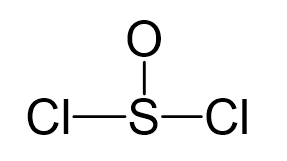There are 6 + 6 + 2×7 = 26 electrons, and 6 are used to make 3 bonds. Two chlorines and the oxygen take 3 lone pairs, and the remaining one goes to the sulfur: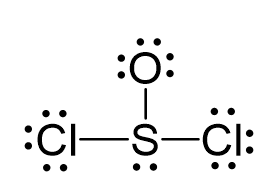All the atoms have an octet, however, there are three lone pairs on the oxygen which gives it a -1 formal charge. Therefore, one of the lone pairs on the oxygen is used to make another bond with the sulfur, and even if it exceeds the octet, that is acceptable for elements in the third row and below because they have d orbitals.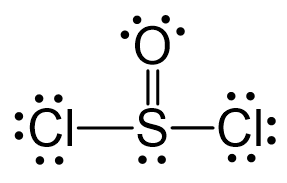The central atom has 3 atoms and a lone pair (SN = 4). Therefore, the electron geometry is tetrahedral while the molecular geometry is trigonal pyramidal: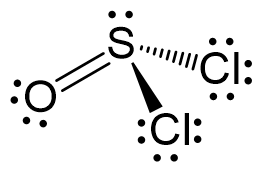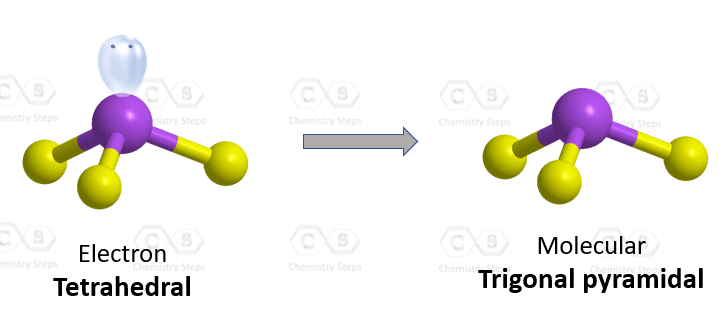Areic number 4 indicates an sp3-hybridization with idealized angles of 109.5o. However, because the groups on the central atom are not identical, the angles are not going to be exactly 109.5o.

Check Also